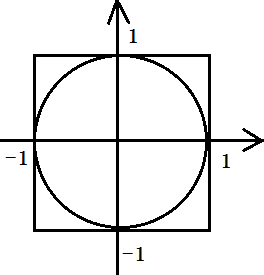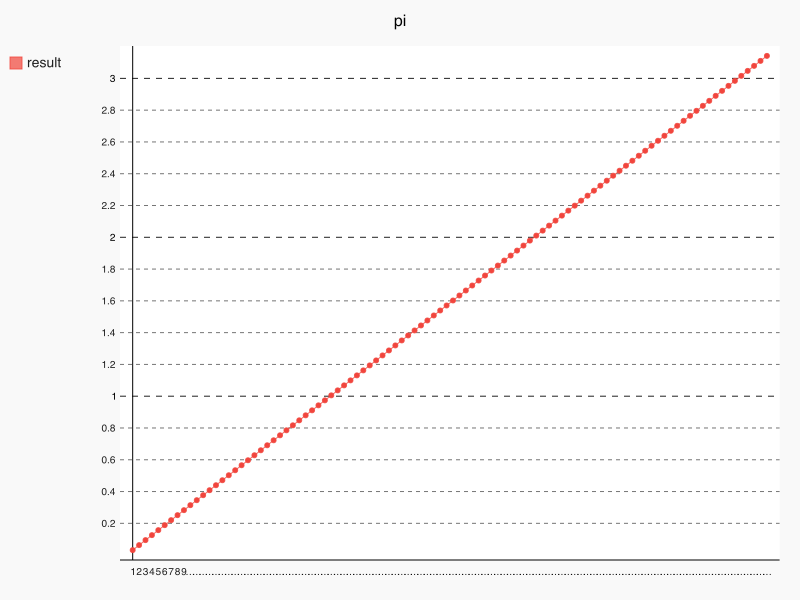# 蒙特卡罗方法

## 一个典型的栗子``````from random import uniform

def pi(times):

hits = 0
for i in range(times):

x = uniform(-1, 1)
y = uniform(-1, 1)

if (x**2 + y**2) - 1 <= 0:
hits += 1
return 4.0 * hits / times``````## 本文链接：

https://jamchoi.me/archives/7.html
1 + 1 =